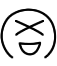# POJ1733 Parity game（带权并查集+离散化）

## Parity game

Time Limit: 1000MSMemory Limit: 65536K
Total Submissions: 10777Accepted: 4139

### Description

Now and then you play the following game with your friend. Your friend writes down a sequence consisting of zeroes and ones. You choose a continuous subsequence (for example the subsequence from the third to the fifth digit inclusively) and ask him, whether this subsequence contains even or odd number of ones. Your friend answers your question and you can ask him about another subsequence and so on. Your task is to guess the entire sequence of numbers.

### Input

The first line of input contains one number, which is the length of the sequence of zeroes and ones. This length is less or equal to 1000000000. In the second line, there is one positive integer which is the number of questions asked and answers to them. The number of questions and answers is less or equal to 5000. The remaining lines specify questions and answers. Each line contains one question and the answer to this question: two integers (the position of the first and last digit in the chosen subsequence) and one word which is either even or odd (the answer, i.e. the parity of the number of ones in the chosen subsequence, where even means an even number of ones and odd means an odd number).

### Output

There is only one line in output containing one integer X. Number X says that there exists a sequence of zeroes and ones satisfying first X parity conditions, but there exists none satisfying X+1 conditions. If there exists a sequence of zeroes and ones satisfying all the given conditions, then number X should be the number of all the questions asked.

### Sample Input

10
5
1 2 even
3 4 odd
5 6 even
1 6 even
7 10 odd

### Sample Output

3

CEOI 1999

### 代码

StatusAccepted
Time32ms
Memory872kB
Length1492
LangG++
#include <iostream>
#include <cstdio>
#include <algorithm>
using namespace std;
const int maxn = 50010;
int F[maxn], sum[maxn], a[maxn], tmp[maxn];
struct Node
{
int l, r;
}node[maxn];

int find(int x)
{
if (x == F[x])
return x;
int t = find(F[x]);
sum[x] = (sum[x] + sum[F[x]]) % 2; // 更新
F[x] = t;
return F[x];
}

int main()
{
int n, m, x, y;
char s;
scanf("%d%d", &n, &m);
int ans = m;
for (int i = 1; i <= (m << 1); ++i)
{
F[i] = i;
sum[i] = tmp[i]= 0;
}
bool flag = true;
int k = 0;
for (int i = 1; i <= m; ++i)
{
scanf("%d%d%s", &node[i].l, &node[i].r, s);
tmp[i] = ((s == 'e') ? 0 : 1);
a[k++] = node[i].l;
a[k++] = node[i].r;
}
sort(a, a + k); // 离散化
int size = unique(a, a + k) - a;
for (int i = 1; i <= m; ++i)
{
x = lower_bound(a, a + size, node[i].l) - a + 1;
y = lower_bound(a, a + size, node[i].r) - a + 1;
x -= 1;
int rootx = find(x), rooty = find(y);
if (rootx != rooty) // 合并
{
F[rooty] = rootx; // 让rootx为父节点
sum[rooty] = (2 + sum[x] - sum[y] + tmp[i]) % 2; // 更新值
}
else
{
if ((2 + sum[y] - sum[x]) % 2 != tmp[i]) // 判断
{
if (flag)
{
ans = i - 1;
flag = false;
}
}
}
}
printf("%d\n", ans);
return 0;
}

WA了两发，没取模加小地方写错。训练还是很有效果的，做的题越多，对知识的理解就越深刻。The end.
2018-04-20 星期五

1.太傅

又复习了一遍离散化！#(吐舌)